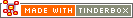^endIf^Code Type: Conditional Mark-up Code Scope of Action: n/a

^endIf^

Indicates the end of the alternatives indicated by the ^if( condition)^ statement. This closing statement is not mandatory if there is no ^else^ branch to the resulting actions. thus the following are acceptable alternatives:

`^if(condition)do stuff^`

`^if(condition)do stuff^^endIf^`

For the next test a closing ^endIf^ is mandatory:

`^if(condition)do stuff^^else^do other stuff^endIf^`

 Up: Scope n/a Previous: ^else^ Next: ^firstWord( data )^

[Last updated: 14 Dec 2009, using v5.0]